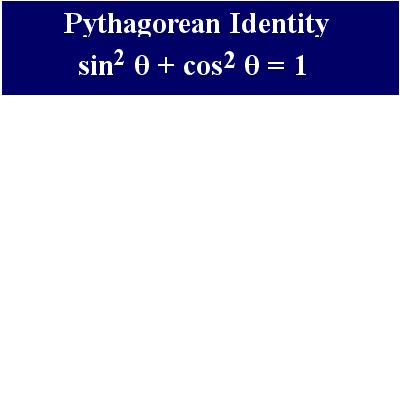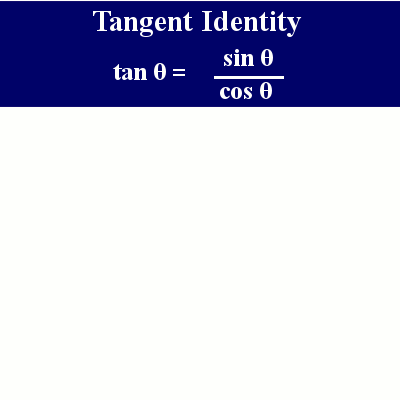# Trig Identities

Back to “Basic Trigonometry” Page

## Pythagorean Identity

Watch the animation below to see how the Pythagorean Identity is really just the Pythagorean Theorem.Use these flashcards to recognize the Pythagorean Identity. The flashcards use (x) instead of θ because the JavaScript for the flashcards does not like the code for θ.

• ﻿sin (x)/sin2 x + cos2x = ; = sin (x)/1 = sin (x)
• sin2 x+ cos2x /sin x = ; = 1/sin x = csc (x)
• 1 - sin2(x) =; = cos2 (x)
• 1 - cos2(x) =; = sin2 (x)

## Tangent Identity

We call it the Tangent Identity. It’s really just one of a bunch of plain old trig identities. But standardized tests like to slip it in.